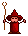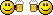Author Topic: Rope physics  (Read 2956 times)r0ber7

• Prof. Inline
••• Posts: 528Rope physics
« on: 2013-Oct-22 »
In an attempt to create more interesting enemies for my game, I decided to figure out how ropes work. Not only could I use that code to, well, hang ropes around the place, I could also make snakes move around. It would also make creating endbosses more interesting and less time-consuming.

Anyway, here's the code. You can download the entire project as a zip file from here.

Code: GLBasic [Select]
// --------------------------------- //
// Project: Ropes
// Start: Tuesday, October 22, 2013
// IDE Version: 10.283
// Robert van Engelen
// www.driewielerdigital.com

// IDENTITY
GLOBAL rope AS p_rope
GLOBAL mx, my, b1, b2

MOUSESTATE mx, my, b1, b2
rope.init( 20, mx, my)

WHILE 1
MOUSESTATE mx, my, b1, b2
rope.play( mx, my)
rope.draw()
WEND

// TYPE
TYPE p_rope

// STATE
str1_x[]        // points
str1_y[]
str2_x[]
str2_y[]
vec_x1          // vectors
vec_x2
vec_y1
vec_y2
at_x1           // points to attach both sides of rope to
at_y1
at_x2
at_y2
segments_n = 10
gravity = 0.0

txt\$            // text to print

// BEHAVIOUR
FUNCTION init : n1, px, py, grav1=1.7, attach_x1=0, attach_y1=0, attach_x2=0, attach_y2=0

self.segments_n = n1
self.gravity = grav1

LOCAL i
LOCAL n = self.segments_n

DIM self.str1_x[n+1]
DIM self.str1_y[n+1]
DIM self.str2_x[n+1]
DIM self.str2_y[n+1]

// initialize points
FOR i=0 TO n-1
self.str1_x[i] = px
self.str1_y[i] = py
//INC self.str1_x[i], 0
INC self.str1_y[i], i*1
NEXT

self.at_x1 = attach_x1
self.at_y1 = attach_y1
self.at_x2 = attach_x2
self.at_y2 = attach_y2

ENDFUNCTION

FUNCTION play : px, py

LOCAL i, n=self.segments_n, normal_length = 3
LOCAL vec_x1, vec_x2, vec_y1, vec_y2, mag1, mag2, ext1, ext2, xv, yv

// set the new top x and y
self.str1_x = px
self.str1_y = py

FOR i=0 TO n-1

STDOUT "I: "+i+"\n"

IF i > 0
vec_x1 = self.str1_x[i-1] - self.str1_x[i]
vec_y1 = self.str1_y[i-1] - self.str1_y[i]
mag1 = SQR( POW(vec_x1, 2) + POW(vec_y1, 2) ) // get the length between two points
ext1 = mag1 - normal_length

STDOUT "ext1: "+ext1+" = "+mag1+"-"+normal_length+"\n"
ENDIF

vec_x2 = self.str2_x[i+1] - self.str2_x[i]
vec_y2 = self.str2_y[i+1] - self.str2_y[i]
mag2 = SQR( POW(vec_x2, 2) + POW(vec_y2, 2) ) // get the length between two points
ext2 = mag2 - normal_length

STDOUT "ext2: "+ext2+" = "+mag2+"-"+normal_length+"\n"

//          xv = (X_Vector1 / Magnitude1 * Extension1) + (X_Vector2 / Magnitude2 * Extension2)
//          yv = (Y_Vector1 / Magnitude1 * Extension1) + (Y_Vector2 / Magnitude2 * Extension2) + Gravity

xv = ( vec_x1 / mag1 * ext1 ) + ( vec_x2 / mag2 * ext2 )
yv = ( vec_y1 / mag1 * ext1 ) + ( vec_y2 / mag2 * ext2 ) + self.gravity

IF vec_x1 = 0 //OR vec_x2 = 0
xv = 0
ENDIF
IF vec_y1 = 0 OR vec_y2 = 0
yv = 0
ENDIF

STDOUT "xv: "+xv+"=("+vec_x1+"/"+mag1+"*"+ext1+"), yv:"+yv+"\n"

self.str2_x[i] = self.str1_x[i] + ( xv*0.5 )
self.str2_y[i] = self.str1_y[i] + ( yv*0.5 )

NEXT

FOR i=0 TO n-1
self.str1_x[i] = self.str2_x[i]
self.str1_y[i] = self.str2_y[i]
NEXT

self.str1_x[n-1] = self.str1_x[n-2]
self.str1_y[n-1] = self.str1_y[n-2]

ENDFUNCTION

// draw stuff
FUNCTION draw :

LOCAL n=self.segments_n

ALPHAMODE -0.6

FOR i=0 TO n-2
DRAWRECT self.str1_x[i]+96, self.str1_y[i], 8, 8, RGB(255,0,0)
DRAWRECT self.str2_x[i]+98, self.str2_y[i], 4, 4, RGB(255,255,0)
NEXT

SHOWSCREEN

ENDFUNCTION

ENDTYPEmentalthink

• Prof. Inline
••• Posts: 3366
• Integrated BrainRe: Rope physics
« Reply #1 on: 2013-Oct-22 »
Very interesting and fun, I played a bit with the code, perhaps in 3D with vertex selection can create a nice FX...
Thanks for sharing the work.